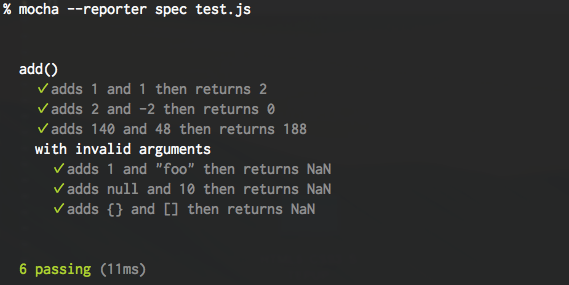mocha-each
Parameterized test utility for MochaLast updated a year ago by ryym .
MIT · Repository · Bugs · Original npm · Tarball · package.json
`\$ cnpm install mocha-each `
SYNC missed versions from official npm registry.

# Mocha-Each - Parameterized Test for Mocha

This module provides a way to write simple parameterized tests in Mocha.

## Installation

``````npm install --save-dev mocha-each
``````

## Usage

### Basic

The function mocha-each provides takes parameters as an array and returns a parameterized test function. You can define test cases for each parameter by the function. All the test cases you defined are executed even if one or more cases fail during the test.

``````// test.js
const assert = require('assert');
const forEach = require('mocha-each');

return parseInt(a) + parseInt(b);
}

forEach([
[1, 1, 2],
[2, -2, 0],
[140, 48, 188]
])
.it('adds %d and %d then returns %d', (left, right, expected) => {
});

context('with invalid arguments', () => {
forEach([
[1, 'foo'],
[null, 10],
[{}, []]
])
.it('adds %j and %j then returns NaN', (left, right) => {
assert(isNaN(value));
});
});
});
``````

Result:### At describe level

Similarly, it works on describe level

``````// test.js
const assert = require('assert');
const forEach = require('mocha-each');

function subtract(a, b) {
return parseInt(a) - parseInt(b);
}

forEach([
[1, 1, 0],
[2, -2, 4],
[140, 48, 92]
])
.describe('subtract() with %d and %d', (left, right, expected) => {
let actual;
before(() => {
actual = subtract(left, right);
});

it('subtracts correctly and returns ' + expected, () => {
assert.equal(actual, expected);
});
});
``````

### Asynchronous code

When testing asynchronous code, you need to add a callback as a last argument of the test function and call it. The callback is same as the one which Mocha's `it` gives. See Mocha - asynchronous code for the detail.

``````forEach([
[0, 1],
[2, 3]
])
.it('does async operation', (arg, expected, done) => {
fetchData(arg)
.then(actual => assert.equal(actual, expected))
.then(done);
});
``````

### Exclusive or inclusive tests

You can call .only() and .skip() in the same way as Mocha's `it`.

``````// Run only these parameterized tests.
forEach([
0, 1, 2, 3
])
.it.only('works fine', number => {
assert(number);
});

// Ignore these parameterized tests.
forEach([
'foo', 'bar', 'baz'
])
.it.skip('also works fine', word => {
assert(word);
});
``````

Note: When you use the `.only()`, mocha-each creates a nameless test suite by describe() to define exclusive parameterized tests because we can't call `.only()` multiple times (see Mocha docs).

## API

### forEach(parameters).it(title, testCase)

#### parameters: `Array`

An array of parameters. Each parameter will be applied to `testCase` as its arguments.

#### title: `String` or `Function`

A title of the test case. You can define each title as a string or function.

##### String title

When it is a string, you can use placeholders which will be replaced by the parameters. mocha-each uses sprintf-js to replace placeholders.

example:

``````forEach([
['Good morning', 9],
['Hello', 12],
['Godd evening', 21]
])
.it('greets "%s" at %d:00', (expected, time) => {
const greet = greeter.at(time).greet();
assert.equal(greet, expected);
});
// =>
// greets "Good morning" at 9:00
// greets "Hello" at 12:00
// greets "Good evening" at 21:00
``````
##### Function title

When it is a function, it takes each parameter and index as its arguments like:

``````  title(p, p, .., p[n - 1], index);
``````

#### testCase: `Function`

A test function which will be called for each parameter. The context of the function is same as Mocha's `it` so that you can use configuration methods like timeout.

``````forEach([
//...
])
.it('is a slow test', function(p0, p1, p2 /*, done */) {
this.timeout(3000); // Configure timeout.
use(p0, p1, p2);
//...
});
``````

## Supported interfaces

Though Mocha provides several interfaces, currently only the BDD interface is supported.

## Tips

You can define the name of mocha-each function as you like when requiring it. Here's a example which uses more BDD-like name.

``````const withThese = require('mocha-each');

describe('findByName()', () => {
withThese([
[1, 'foo'],
[2, 'bar'],
[3, 'baz']
])
.it('should find data by name', (id, name) => {
const data = findByName(name);
assert.equal(data.id, id);
});
});
``````

MIT

## Current Tags

• 2.0.1                                ...           latest (a year ago)

## 9 Versions

• 2.0.1                                ...           a year ago
• 2.0.0                                ...           a year ago
• 1.2.0                                ...           a year ago
• 1.1.0                                ...           3 years ago
• 1.0.5                                ...           3 years ago
• 1.0.3                                ...           4 years ago
• 1.0.2                                ...           4 years ago
• 1.0.1                                ...           4 years ago
• 1.0.0                                ...           4 years ago